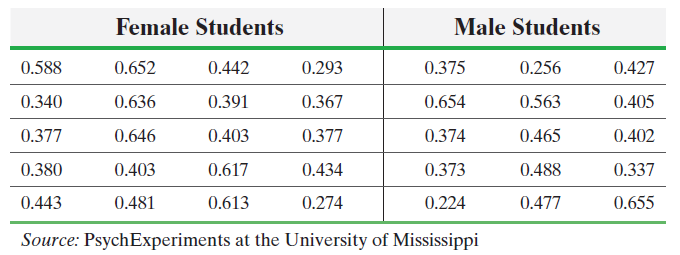×
Get Full Access to Statistics: Informed Decisions Using Data - 5 Edition - Chapter 11.3 - Problem 14
Get Full Access to Statistics: Informed Decisions Using Data - 5 Edition - Chapter 11.3 - Problem 14

×

# ?Measuring Reaction Time Researchers wanted to determine whether the reaction time (in seconds) of males differed from that of females to a go/no go stISBN: 9780134133539 240

## Solution for problem 14 Chapter 11.3

Statistics: Informed Decisions Using Data | 5th Edition

• Textbook Solutions
• 2901 Step-by-step solutions solved by professors and subject experts
• Get 24/7 help from StudySoup virtual teaching assistantsStatistics: Informed Decisions Using Data | 5th Edition

4 5 1 270 Reviews
24
4
Problem 14

Measuring Reaction Time Researchers wanted to determine whether the reaction time (in seconds) of males differed from that of females to a go/no go stimulus. The researchers randomly selected 20 females and 15 males to participate in the study. The go/no go stimulus required the student to respond to a particular stimulus and not to respond to other stimuli. The results are as follows:(a) Is it reasonable to use Welch’s t-test? Why? Note: Normal probability plots indicate that the data are approximately normal and boxplots indicate that there are no outliers.

(b) Test whether there is a difference in the reaction times of males and females at the= 0.05 level of significance.

(c) Draw boxplots of each data set using the same scale. Does this visual evidence support the results obtained in part (b)?

Step-by-Step Solution:

Step 1 of 5) Measuring Reaction Time Researchers wanted to determine whether the reaction time (in seconds) of males differed from that of females to a go/no go stimulus. The researchers randomly selected 20 females and 15 males to participate in the study. The go/no go stimulus required the student to respond to a particular stimulus and not to respond to other stimuli. The results are as follows: (a) Is it reasonable to use Welch’s t-test Why Note: Normal probability plots indicate that the data are approximately normal and boxplots indicate that there are no outliers. (b) Test whether there is a difference in the reaction times of males and females at the = 0.05 level of significance. (c) Draw boxplots of each data set using the same scale. Does this visual evidence support the results obtained in part (b) If we reject, H0: m1 = m2, we can look at the bounds of the confidence interval to determine which mean (m1 or m2) is greater. For example, if the interval contains only positive numbers, then x1 - x2 is positive, so we have evidence that m1 7 m2.

Step 2 of 2

## Discover and learn what students are asking

Statistics: Informed Decisions Using Data : Testing the Significance of the Least-Squares Regression Model
?In Problems 5–10, use the results of Problems 7–12, respectively, from Section 4.2 to answer the following questions: (a) What are the estima

Unlock Textbook Solution